Courses

XAT Quantitative Ability And DI MCQ Quiz - 2

29 Questions MCQ Test MBA Exams Mock Test Series and Past Year Question Papers | XAT Quantitative Ability And DI MCQ Quiz - 2

Description
Attempt XAT Quantitative Ability And DI MCQ Quiz - 2 | 29 questions in 60 minutes | Mock test for CAT preparation | Free important questions MCQ to study MBA Exams Mock Test Series and Past Year Question Papers for CAT Exam | Download free PDF with solutions
QUESTION: 1

There are five identical green dyes, four identical blue dyes, three identical yellow dyes and four different red dyes. How many combinations of dyes can be chosen taking at least one green, at least one blue and at least one red dye?

Solution:

For Green dyes: Since at least one green dye has to be selected, we can do so in 5 ways by selecting either 1,2, 3, 4 or all 5 green dyes. Since the dyes are identical, it does not matter which dye is selected. Thus, only the count of dyes leads to possibilities.
For Blue dyes: 4 ways (Similar calculation as in the case of Green dyes) For Yellow dyes: In case of yellow dyes, there is no ‘atleast’ constraint, thus we can select yellow dyes in 3 + 1 = 4 ways by selecting either 0, 1, 2 or all 3 dyes. This means that we might have a selection in which there is not even one yellow dye.

For Red dyes: Red dyes are all different from each other. Thus in this case, it matters which dye is selected and also how many dyes are selected. Thus, for every dye we have two options - either select it or leave it. Thus for each of the 4 red dyes we have 2 possibilities each, leading to a total of 2 x 2 x 2 x 2 = 24 ways.

But these 24 ways include a possibility in which none of the red dyes is selected. But we want at least one red dye in our selections. Hence we subtract this possibility from the total. 24 - 1 =15 ways The total number of ways = 5 x 4 x 4 x (24 - 1) = 1200 Hence, option 3.

QUESTION: 2

Three friends - Annie, Bobby and Carole like to play with numbers. Annie picks a random natural number x, triples it and gives the result to Bobby. Each time Bobby gets a number from Annie; he adds 75 to the number and gives the result to Carole. Each time Carole gets a number from Bobby, she doubles the number and subtracts 50 from it and gives the result to Annie. Annie again triples it and gives the result to Bobby and this process continues. The first person to receive a 5-digit number (from other) is declared the winner. If Bobby wins the game, what is remainder when the least possible value of x is divided by 13?

Solution:

From the given data,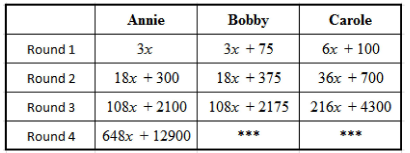Bobby wins the game.

⇒Bobby received a 5-digit number. 108x+2100 >9999 ⇒ x = 74 Remainder when 74 is divided by 13 = 9 Hence, option 3.

QUESTION: 3

Group Question Answer the following question based on the information given below. Rakesh, Rajesh, Rupesh, Paresh and Suresh were hired by Glotel information as sales officers to sell simcards on a contract basis. For each simcard sold on day 1 and day 2, each sales officer was given Rs. 25; and an amount of Rs. 5 was deducted for each unsold simcard from the amount they had earned in the day. On the 1st day, each sales officer was given 25 simcards to sell. On the 2nd day, each sales officer was allowed to sell any number of simcards from Glotel information at the cost of Rs. 5 per simcard, from the money earned on day 1.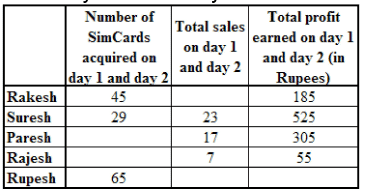Q. How many simcards did Rajesh sell on day 1?

Solution:

Each sales officer got 25 simcards to sell on day 1.
If a sales officer sold x number of simcards on day 1, then the profit earned by him on day 1 is: = 25x - 5(25 - x) = 30x - 125 Let y be the number of simcards acquired by Rajesh to sell on day 2.

Since Rajesh sold 7 simcards on day 1 and day 2, the number of simcards sold by him on day 2 will be (7 - x).
Profit earned by him on day 2 = [25(7 - x) - 5 { y - (7 - x)}] And the total profit earned by him = Rs. 55. Therefore  ( 30 x - 125 - 5y) + [25(7 - x) - 5 { y - (7 - x)}] = 55. So  30 x - 125 - 5y + 175 - 2 5 x - 5 y + 35 - 5x = 55
⇒y = 3

The number of simcards Rajesh bought on day 2 is 3, that means Rajesh could have sold at most 3 simcards on day 2.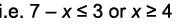Rajesh could have sold 4, 5, 6 or 7 simcards on day 1. Hence, option 5.

QUESTION: 4

Rakesh, Rajesh, Rupesh, Paresh and Suresh were hired by Glotel information as sales officers to sell simcards on a contract basis. For each simcard sold on day 1 and day 2, each sales officer was given Rs. 25; and an amount of Rs. 5 was deducted for each unsold simcard from the amount they had earned in the day. On the 1st day, each sales officer was given 25 simcards to sell.
On the 2nd day, each sales officer was allowed to sell any number of simcards from Glotel information at the cost of Rs. 5 per simcard, from the money earned on day 1.Q. Which of the following may not be the profit for Rakesh on day 1?

Solution:

Rakesh acquired 20 simcards to sell on day 2.
Let Rakesh sell y simcards on day 2. (30x - 125 - 100 + 25y - 5(20 - y)) = 185. 30x + 30y = 510 ⇒ x + y = 17
We know that 3 0x - 125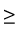100 as simcards are bought on day 2 using the money earned on day 1.

Therefore x7.5.

x can be 8, 9, 10, 11, 12, 13, 14, 15, 16 or 17.
The profit for Rakesh on day 1 could be, 3 0 x - 125 = Rs. 115, Rs. 145, Rs. 175, Rs. 205, Rs. 235, Rs. 265, Rs. 295, Rs. 325, Rs. 355 and Rs. 385 Hence, option 2.

QUESTION: 5

Rakesh, Rajesh, Rupesh, Paresh and Suresh were hired by Glotel information as sales officers to sell simcards on a contract basis. For each simcard sold on day 1 and day 2, each sales officer was given Rs. 25; and an amount of Rs. 5 was deducted for each unsold simcard from the amount they had earned in the day. On the 1st day, each sales officer was given 25 simcards to sell.
On the 2nd day, each sales officer was allowed to sell any number of simcards from Glotel information at the cost of Rs. 5 per simcard, from the money earned on day 1.Q. What is the total number of simcards acquired by all 5 sales 0fficers to sell on day 1 and day 2?

Solution:

As explained in the first question, Rajesh bought a total of 28 simcards on day 1 and day 2.
Let Paresh have sold x simcards on day 1 and y be the number of simcards acquired by Paresh on day 2, then

(3 0x - 125 - 5y) + [25(17 - x) - 5 { y - (17 - x)}] = 305. Therefore 30x - 125 - 5y + 425 - 25x - 5y + 85 - 5x = 305
⇒y = 8
The total number of simcards acquired by Paresh on day 2 is 8.
The total number of simcards acquired by Paresh on day 1 and day 2 is 25 + 8 = 33

The total number of simcards acquired by all 5 sales officers to sell on day 1 and day 2 = 45 + 29 + 33 + 28 + 65 = 200 Hence, option 3.

QUESTION: 6

Rakesh, Rajesh, Rupesh, Paresh and Suresh were hired by Glotel information as sales officers to sell simcards on a contract basis. For each simcard sold on day 1 and day 2, each sales officer was given Rs. 25; and an amount of Rs. 5 was deducted for each unsold simcard from the amount they had earned in the day. On the 1st day, each sales officer was given 25 simcards to sell.
On the 2nd day, each sales officer was allowed to sell any number of simcards from Glotel information at the cost of Rs. 5 per simcard, from the money earned on day 1.Q. If the total profit for Rupesh was the highest among the 5 sales  officers, then what is the minimum number of simcards sold by Rupesh on day 1 and day 2?

Solution:

Rupesh acquired 40 simcards to sell on day 2.
Let Rupesh sell y simcards on day 2, then the total profit for Rupesh is, [30x - 1 2 5 - 2 0 0 + 25y - 5(40 - y)] > 525,

30x - 125 - 200 + 25y - 200 + 5 y > 525 30x + 30y > 1050 ⇒ x + y > 35
The minimum number of simcards sold by Rupesh on day 1 and day 2 is 36.
Hence, option 2.

QUESTION: 7

Rakesh, Rajesh, Rupesh, Paresh and Suresh were hired by Glotel information as sales officers to sell simcards on a contract basis. For each simcard sold on day 1 and day 2, each sales officer was given Rs. 25; and an amount of Rs. 5 was deducted for each unsold simcard from the amount they had earned in the day. On the 1st day, each sales officer was given 25 simcards to sell.
On the 2nd day, each sales officer was allowed to sell any number of simcards from Glotel information at the cost of Rs. 5 per simcard, from the money earned on day 1.Q. If Paresh sold 12 simcards on day 1, then what is the  difference between the number of unsold simcards on day 1 and day 2 for Paresh?

Solution:

As seen in the answer to the third question of this set, Paresh acquired 8 simcards on day 2.
Paresh sold 17 - 12 = 5 simcards on day 2 The unsold simcards for Paresh on day 2 = 8 - 5 = 3 The difference between the unsold simcards on day 1 and day 2 for Paresh: = 13 - 3 = 10
Hence, option 4.

QUESTION: 8

Which of the following is true?

Solution: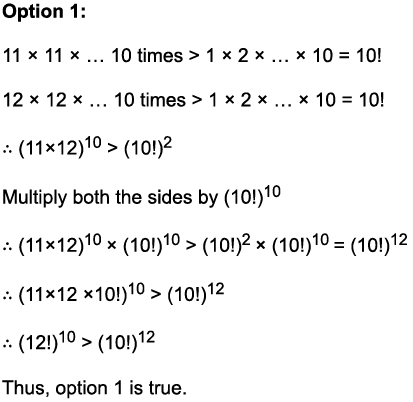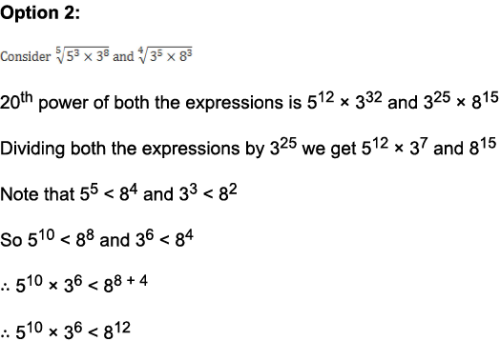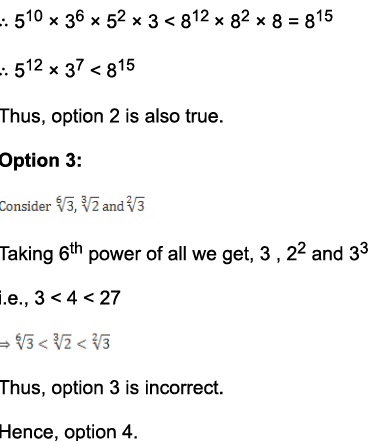QUESTION: 9

Group Question

Answer the following question based on the information given below.

Andy buys four articles, A, B, C, D for a sum of Rs x. He uses A and sells the rest to Bob for Rs. x. Bob uses B and sells the rest to Charlie for Rs. x. Charlie uses C and sells D to David for Rs.x. It is known that Andy, Bob and Charlie made a profit of 20%, 25% and 50% respectively. Also x = 100.

Q. How much did Charlie earn?

Solution:

Let the cost price of A, B, C and D for Andy be a1, b1, c1 and d1 respectively.
Let the cost price of B, C and D for Bob be b2, c2 ancl d2 respectively.
Let the cost price of C and D for Charlie be C3 and d3 respectively.
Then, as per conditions, a1 + b1 + c+ d= 100. b2 + c2 + d2 = 100, C3 + d3 = 100 Therefore Andy’s profit = a1 .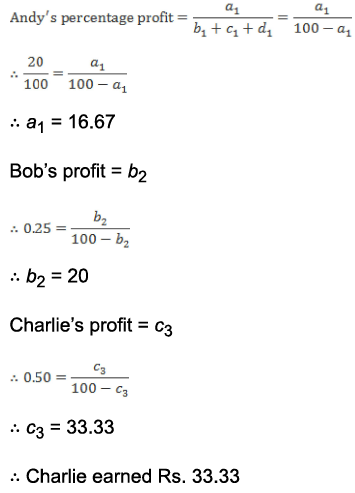Hence, option 3.

QUESTION: 10

Andy buys four articles, A, B, C, D for a sum of Rs x. He uses A and sells the rest to Bob for Rs. x. Bob uses B and sells the rest to Charlie for Rs. x. Charlie uses C and sells D to David for Rs.x. It is known that Andy, Bob and Charlie made a profit of 20%, 25% and 50% respectively. Also x = 100.

Q. For how much more than Andy did Bob buy B, C and D?

Solution:

From the answer to the first question of this set, Andy bought B, C and D for 100 - a1 Bob bought B, C and D for 100.
So, extra amount that Bob paid - a1 = 16.67 Hence, option 2.

QUESTION: 11

Ajay and Suresh are playing a game of dice. Suresh, being the evil one, loaded the dice in such a way that the probability of getting any number n is n times the probability of showing up of 1 when the dice is rolled. The rules of the games are such that for every time an odd number shows up, Ajay scores a point, otherwise the point goes to Suresh. What is the probability of Ajay scoring a point at any single roll?

Solution:

If the probability of getting 1 = P(1) = x, then as per the conditions of loading of the dice, P(2) = 2x, P(3) = 3x and so on.
Now, since getting the numbers 1 or 2 or... or 6 are mutually exclusive and collectively exhaustive events, the sum of all their probabilities will be 1.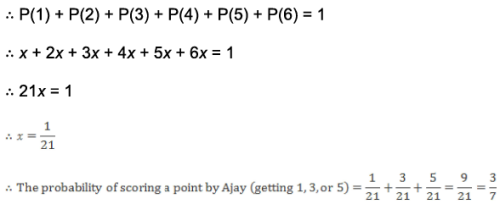Hence, option 2.

QUESTION: 12

Given that k = 2 - (|x - 2| + |x + 2.4|). Find the number of integral values of x for which k takes the maximum possible value.

Solution:

Let f(x) = |x - 2| + |x + 2.4|

Now if we want to maximize k we should look to minimize f(x). By taking values with (x < -2.4), (-2.4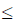x2) and (x > 2) we see that f(x) is minimum in the range (-2.4x2). That is because f(x) is nothing but the sum of distances of x from the points -2.4 and 2 on the number line. This sum of distances is minimum when x is between -2.4 and 2. If (x < -2.4) or (x > 2), then the distance from one of -2.4 or 2 goes on increasing thus increasing the value of f(x).
Lets confirm by taking values,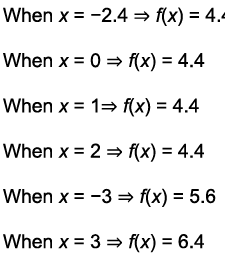Thus as we move away from x = -2.4 or from x = 2, f(x) starts increasing in value. But in the range (-2.4x2), f(x) is at its minimum and is constant at 4.4 Thus x can take 5 integral values (-2, -1, 0, 1,2) in the range (-2.4x2) such that f(x) is minimum. This means for 5 values of x, k attains its maximum value.
Hence, option 4.

QUESTION: 13

Baban Narang, a shooting expert, once decides to practice his shooting skills by targetting a wall clock. His bullet takes 10 seconds to travel to the clock after leavng his rifle. Just before he fires the bullet, the clock shows 5:30:00 pm. What is the probability that the bullet strikes in the smaller angle made between the minute hand and the second hand? Assume that the bullet definitely hits the clock with all points on the clock being equally likely targets.

Solution:

Initial angle between the minute hand and the second hand is,

(30/60)x 360 = 180

Angular velocity of second hand = 360° per minute .Angular velocity of the minute hand = 6° per minute. Relative angular velocity = 354° per minute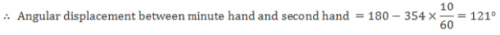Probability that the bullet strikes within the smaller angle made by the minute hand and the second hand is 121/360.
Hence, option 1.

QUESTION: 14

Group Question

Answer the following question based on the information given below.

The following graphs give annual data of the net income, profit (as percentage of net income) and bonus provided to the employees (as percentage of the profit) of a company during the period 2006 - 2010.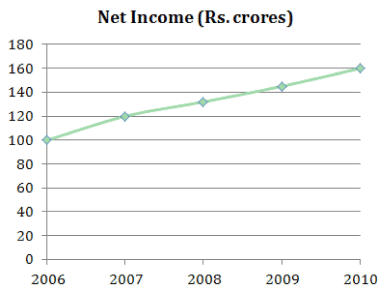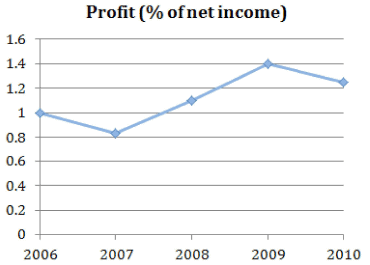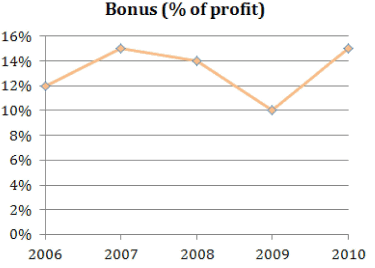Q. The company has 1000 employees in the year 2008 and 1200 employees in the year 2010. By what approximate percentage did the average bonus received by each employee increase in 2010 over 2008?

Solution:

The net income of the company in 2008 and in 2010 is Rs. 130 crores and Rs. 160 crores respectively.
The company registered profits of 1.1% and 1.25% in 2008 and 2010 respectively and the bonus paid was 14% and 15% respectively.
Hence, bonus given by the company,

in 2008 = 14% of 1.1% of 130 crores = 2002 x 10-4 crores in 2010 = 15% of 1.25% of 160 crores = 3000 x 10-4 crores Hence, average bonus received by each employee, in 2008 = Rs. 2002 x 10-4 x 107/1000 = Rs. 2002, and in 2010 = Rs. 3000 x 10-4 x 107/1200 = Rs. 2500 Average bonus received by each employee increases by 498 x 100/2002 ⇒ 25% Hence, option 2.

QUESTION: 15

The following graphs give annual data of the net income, profit (as percentage of net income) and bonus provided to the employees (as percentage of the profit) of a company during the period 2006 - 2010.Q. What is the approximate percentage of the total net income that was given away as bonus by the company during the period 2006 to 2010?

Solution:

Total net income during the period 2006 to 2010 is equal to (100 + 120 + 130 + 145 + 160) = 655 crores.
In the year 2006, the profit is 1% of the net income (Rs. 100 crores).
Profit (in terms of Rs. crores) = Rs. 1 crore.
Now, company gave 12% of the profit as a bonus in 2006.
Hence, bonus given by the company in 2006 = 12% of 1 crore = 12 lakhs.
Similarly, the profit and the bonus given by the company can be calculated and it is tabulated in the following table.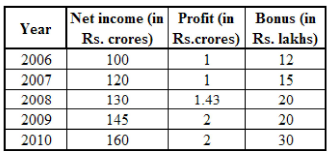From the above table, we get, Total bonus given to the employees during the period 2006 to 2010 = (12 + 15 + 20 + 20 + 30) = Rs. 97 lakhs. = 0.97 crores. Approximate percentage = 0.97 * 100/655 ⇒ 0.148%
Hence, option 2.

QUESTION: 16

The following graphs give annual data of the net income, profit (as percentage of net income) and bonus provided to the employees (as percentage of the profit) of a company during the period 2006 - 2010.Q. If the average bonus received by each employee remained constant in the years 2006 and 2007, then by what percentage did the number of employees increase in 2007 as compared to that in 2006?

Solution:

From the solution of previous question, we get that the company gave Rs. 12 lakhs and Rs. 15 lakhs as a bonus in the year 2006 and 2007 respectively.
Let n-1 be the number of employees in the year 2006 and n2 be the number of employees in the year 2007

Therefore 1200000/n1 = 1500000/n2

n: n2 = 4 : 5

Suppose that there are 4k employees in the year 2006, hence there were 5k employees in 2007, where k is any natural number.
Thus percentage increase in the number of employees in the year 2007 as compared to that in 2006 was, (5k - 4k) x 100/4k =25% Hence, option 3.

QUESTION: 17

The following graphs give annual data of the net income, profit (as percentage of net income) and bonus provided to the employees (as percentage of the profit) of a company during the period 2006 - 2010.Q. The net income of the company increases by 25% in 2011 as compared to that in 2010, and each of the 1000 employees receives the bonus of Rs. 4500 in 2011. What is the percentage increase in the amount of profit earned by the company in 2011 as compared to that in 2010, if it is given that the company had distributed 15% of the profit earned in 2011 as bonus?

Solution:

Total bonus distributed among 1000 employees = 4500 x 1000 = Rs. 4500000 This is 15% of the total profit earned in 2011. Profit earned in 2011 = 4500000 x 100/15= Rs. 3 crores.
From solution of second question, we get that the profit earned by the company in 2010 was 2 crores. Percentage increase in the amount of profit earned = (3 - 2) x 100/2 = 50% Hence, option 2.
Note: Calculating the net income of the company in 2011 is redundant in this question.

QUESTION: 18

Each question is followed by two statements, A and B. Choose the most appropriate options given below.

In trapezium ABCD, AB || CD, and AB = 2 and BC = 8. Angle bisector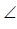ADC meets BC in E. What is the area of trapezium ABCD?

A. E is the midpoint of BC.

Solution:

To find the area of a trapezium, we need the length of its parallel sides and its height.
Considering statement A alone: Following diagram depicts this scenario.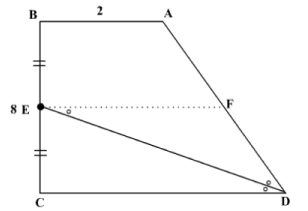Let’s draw EF parallel to DC.
As E is the mid-point of BC, F must also be mid-point of AD.
Now,FED =EDC =EDF Hence, triangle EFD is an isosceles triangle.
Hence, EF = FD = AD/2 But we don't know AD. Hence, we cannot compute FD or CD. Hence, statement A alone is not sufficient to answer the question. Considering statement B alone: In this case diagram will look like this.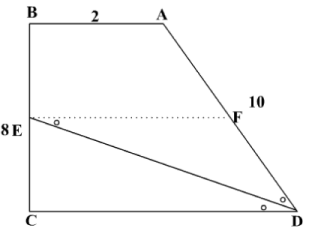We don't get any information to compute the area of trapezium. Hence, statement II alone is not sufficient to answer the question.
Considering statements A and B together: Length of one of the parallel sides is given. So, we need to find length of other parallel side and height.
Given information can be depicted in the following diagram.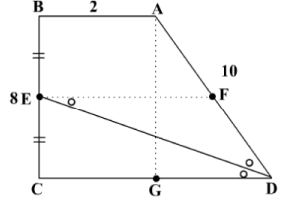Here, line EF is drawn parallel to side CD and line AG is drawn parallel to side BC.
As explained above, EF = DF = AF = 10/2 = 5
Now, in trapezium ABCD, EF is the median of the trapezium. So, EF = (1/2) x (AB + CD). CD = 8

Now we need to find the height of the trapezium.
As, AG is drawn parallel to BC, ABCG is a parallelogram.
So, AG = 8 and CG = 2. GD = 6
In triangle AGD, AD = 10, AG = 8 and GD = 6. AD2 = AG2 + GD2
AGD is a right angled triangle.
So AG is the height of the trapezium.
As we know the length of parallel sides and height of the trapezium, we can compute the area of trapezium.
Hence, the question can be answered by using both the statements.
Hence, option 4.

QUESTION: 19

The teacher asked a class of fifth grade students to find the average of 15 different two-digit numbers displayed on the blackboard. After the students finished the exercise, the teacher wrote the correct answer on the blackboard. Ankit, in an attempt to impress the teacher, claimed that he could reverse the digits of one of the numbers so that the new number also was a two-digit number, and the average he would get would be 2.4 more than the result displayed.

What is the probability of Ankit finding such a number on the blackboard?

Solution:

Since average of the fifteen numbers has been increased by 2.4, therefore the sum of the fifteen numbers Ankit has on his notebook must be greater than the actual sum by 15 x 2.4 = 36 Since, Ankit has left all the other fourteen numbers unaltered, that means that the new number formed by reversing the digits on the original number is greater than the original number by 36.
Let’s say that the original number was XY, therefore the new number will be YX.
Therefore, (10Y + X) - (10X+ Y) = 36 or, 9 ( Y - X ) = 36 or, Y - X = 4

Therefore, any number which does not have zero as one of its digits (the number cannot have zero since any number having zero when reversed will be turned to a one digit number) and where the difference of the digits is 4 will help Ankit to achieve his feat.
15, 26, 37, 48, 59 are the required numbers that can help Ankit.
Number of two digits numbers possible = 90 Number of ways in which 15 two digits number can be selected = 90C15
Number of ways in which 15 numbers can be selected such that the five numbers that can help Ankit in performing the mischief are not selected = 85C15 Hence, the probability that Ankit will find one of the given five numbers on the blackboard = 1 - (85C15/ 90C 15) Hence, option 4.

QUESTION: 20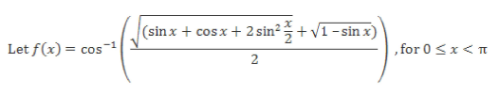What is the sum of all roots of f(x)?

Solution:

Roots are the values of x for which f(x) becomes 0.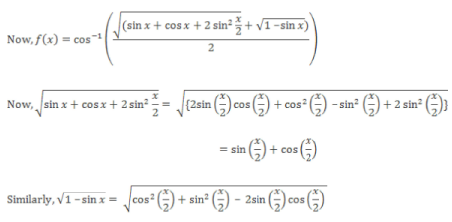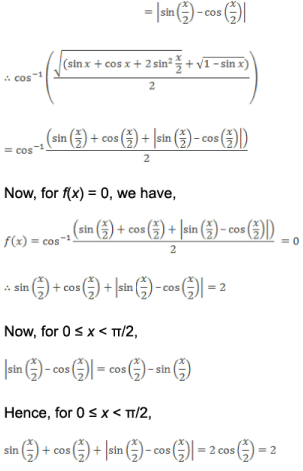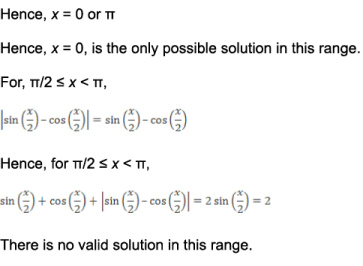Hence, sum of all possible roots = 0 Hence, option 4.

QUESTION: 21

There is a set, A of six whole numbers having mean 16. Few facts about the numbers of A are: 12 is the only mode and the median is 15. What is the minimum possible value of the largest number from this set?

Solution:

Mean of the six numbers = 16.Sum of the six numbers = 16 * 6 = 96 Median = 15 Sum of the 3rd and the 4th number = 30 As 12 is the only mode, 12 must appear at least twice in the set.
If 12 appears twice, sum of the first four numbers < 54 If 12 appears thrice, sum of the first four numbers = 54 Thus, the maximum sum of the first four numbers = 54 In order to minimize the largest number, sum of the last two numbers must be the least possible i.e., the sum of the first four numbers must be maximum.
The sum of the last two numbers = 96 - 54 = 42.42= 21 +21 = 20 + 22 = ... and so on.
If 21 appear in set A, it must have appeared twice. As 12 is the only mode, it must have appeared thrice.
Thus, A = {12, 12, 12, 18,21,21} The minimum possible value of the largest number = 21 Hence, option 2.

QUESTION: 22

Group Question

Answer the following question based on the information given below.

The graph below shows the total domestic sales of three detergent brands (in Rs. crores) over a period of six years.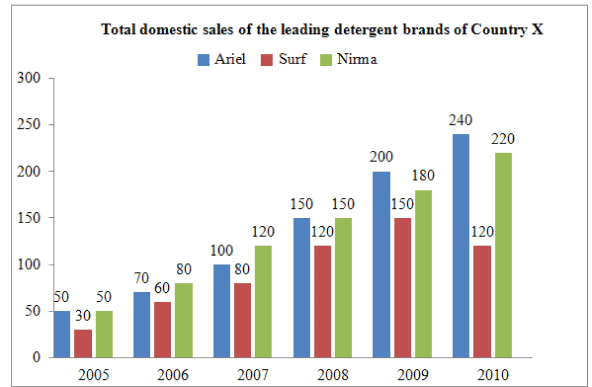Q. Over the entire period, what is the maximum average annual  growth rate achieved by any of these three brands?

Solution:

The average annual growth for any of these brands is equal to the arithmetic mean of the growth rate calculated on a year-on-year basis for that particular brand.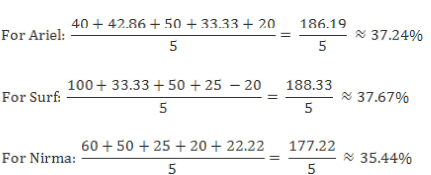Thus, the maximum average annual growth rate over this period is achieved by Surf and it is 37.67%.
Hence, option 3.

QUESTION: 23

The graph below shows the total domestic sales of three detergent brands (in Rs. crores) over a period of six years.Q. For any detergent brand in country X, if the exports (in Rs. crores) in a particular year do not exceed the domestic sales for that year, which of these statement(s) is/are definitely true?

I. The exports of Surf can exceed the average domestic sales of Ariel in at most two years.
II. The exports of Nirma in 2010 are more than the total exports of Ariel in 2005 and 2006.
III. The exports of Surf in 2007 cannot be equal to the maximum value of its exports in 2008.

Solution:

The exports in a certain year do not exceed the domestic sales for that year. i.e. ExportsDomestic Sales.
Consider statement II.
The maximum exports of Nirma in 2010 are Rs. 220 crores and the maximum exports of Ariel in 2005 and 206 are 50 + 70 i.e. Rs. 120 crores.
However, the actual exports of Nirma in 2010 can take any value from 0 to Rs. 220 crores.
Hence, statement II may or may not be true.
Consider statement I.

Average domestic sales of Ariel = (50 + 70 + 100 + 150 + 200 + 240)/6 = Rs. 135 crores.
Since ExportsDomestic Sales, the exports of Surf can be more than 135 crores only in 2009 (where the domestic sales are Rs. 150 crores).
Hence, the exports of Surf can exceed the average domestic sales of Ariel in at most one year.
Hence, statement I is not true.
Consider Statement III: mThe maximum exports of Surf in 2007 can be Rs. 80 crores while the maximum exports of Surf in 2008 can be Rs. 120 crores.
So, irrespective of what the actual exports of Surf in 2007 are, they can never be equal to the maximum exports of Surf in 2008. Hence, statement III is true.
Hence, option 2.

QUESTION: 24

The graph below shows the total domestic sales of three detergent brands (in Rs. crores) over a period of six years.Q. An analyst working on these figures erronously applied the growth rate of Surf from 2008 to 2009 to Ariel for the same period. However, he applied the correct growth rates every where else. What value did he get (in Rs. crores) for domestic sales of Ariel in 2010?

Solution:

The growth rate of Surf from 2008 to 2009 is 25%. So, the analyst erroneously applied this value to Ariel from 2008 to 2009. However, he used the correct value for Ariel in 2009-10 i.e. 20%.
Domestic sales for Ariel in 2010 (in Rs. crores) = 150 * 1.25 x 1.2 = 225 Hence, option 5.

QUESTION: 25

Each question is followed by two statements, A and B. Choose the most appropriate options given below.

Roots of x3 + ax2 + bx + c = 0 are integers and are in arithmetic progression. If a, b, and c are also integers, then what is the value of a + b + c?

A. a2 = 3(b + 4) and 4a < b < 6a

B. 5a = b + 4 and c = 7a

Solution:

As roots of the equation are in A.R, hence let the three roots be p - r, p and p + r.
Here p and r are integers.
Now, p - r + p + p + r = - a. a = - 3p, b = p(p - r) + p(p + r) + (p - r)(p + r) = 3p2 - r2. c = - p( p2 - r2 )

Considering statement A alone: a2 = 3 (b + 4). 9p2 = 3(3p2 - r2 + 4).
r= 2
Also, as a2 is multiple of 3 and a is an integer, hence a is also a multiple of 3.

Also, 4a < b < 6a Multiplying the expression by 3 and adding 12,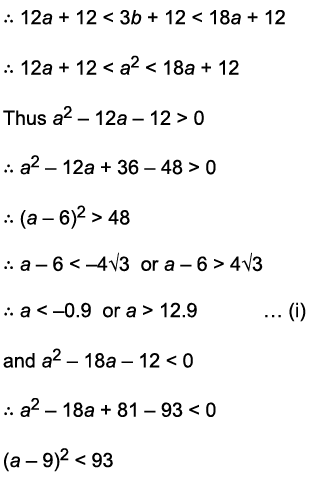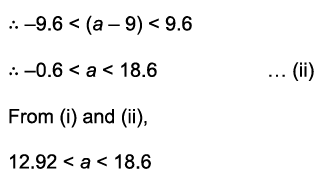Now, a is a multiple of 3, hence we can conclude that a can either be 15 or 18.
Correspondingly, p = -5 or p = -6 We get two corresponding values of (a + b + c).
Statement A alone is insufficient to solve the question.

Consider statement B alone: 5a = b + 4 and c = 7a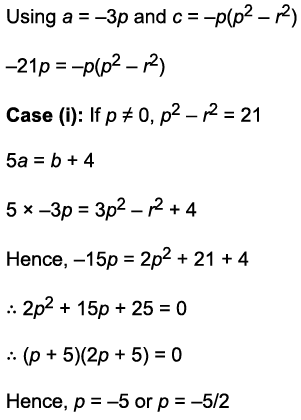But p is integer, hence p = -5 is the only valid solution.
Using, p2 - r2 = 21, r = 2. The value of (a + b + c) corresponding to p = -5 and r= 2 can be found.
Case (ii): If p = 0, a = 0, b = -4, r = 2 and hence a + b + c can be found.
However, we find two values of (a + b + c).
Hence, statement B alone is also not sufficient to answer the question.
Considering both statements A and B together: p = - 5 and r - 2. A unique value of (a + b + c) corresponding to this can be found.
Hence, option 4.

QUESTION: 26

There are 6 straight lines in a plane, no two of which are parallel and no three of which pass through the same point. If their points of intersection are joined, then the number of additional lines thus introduced is:

Solution:

6 straight lines are in a plane, no two of which are parallel and no three of which pass through the same point.
Thus it follows that each line will intersect with every other line.
Since for every intersection point, 2 lines are required, 6C2 = 15 points gives us the total number of unique intersection points.
Also, since each line intersects every other line, there are 5 intersection points on each line and these 5 intersection points are collinear.

Let us consider any one intersection point out of the 15 points obtained above.
In order to calculate the number of additional lines through this point we will have find out how many intersection points are collinear with the point in question.
Since the intersection point lies on two intersecting lines, we infer that it is collinear with the 4 other intersection points on each of the two lines.
Hence, in total it is collinear with 4 + 4 = 8 points. Thus additional lines can be drawn with the remaining 6 intersection points, i.e. 6 additional lines can be drawn from each intersection point.

Total additional lines = (15 x 6)/2  (Since lines are being counted twice) = 45
Hence, option 2.

QUESTION: 27

A regular hexagon is inscribed in a circle of radius 7 cm. Inside the hexagon a rectangle is inscribed such that it touches the hexagon at the midpoints of its sides. A circle, whose centre coincides with that of the outer circle, is inscribed in the rectangle and an equilateral triangle is inscribed in the smaller circle. What is the length of the side of the triangle?

Solution: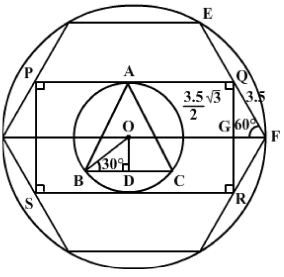Radius of the circle is 7 cm. Side of the hexagon is 7 cm. Quadrilateral PQRS is drawn in the hexagon such that its vertices touch the midpoints of sides of the hexagon.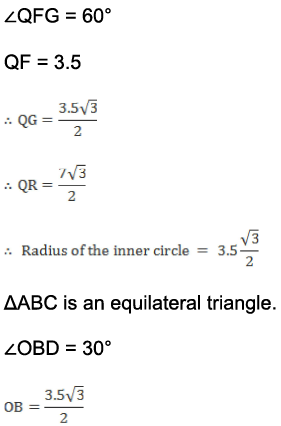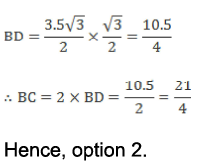QUESTION: 28

Which condition should hold true if the equation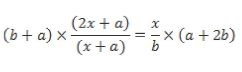has real roots that are equal in magnitude but opposite in sign?

Solution:

Re-arranging the terms we get,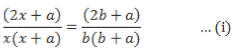By observation, we can easily identify that one of the roots of the above equation is x = b Thus, since the roots are equal in magnitude and opposite in sign, the other root must be x = -b Thus substituting x = -b in (i) we get,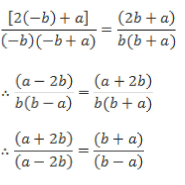By Componendo-dividendo we get,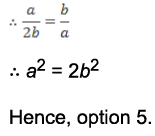QUESTION: 29

If (1 - 2x) * log (2.5m) = (x - 1) * log m, then which of the following best describes x?

Solution: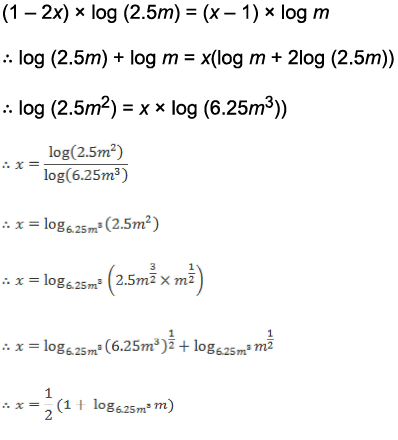Hence, option 2.Use Code STAYHOME200 and get INR 200 additional OFF Use Coupon Code

How to Prepare for CAT

Read our guide to prepare for CAT which is created by Toppers & the best Teachers

Track your progress, build streaks, highlight & save important lessons and more!

Similar ContentRelated tests